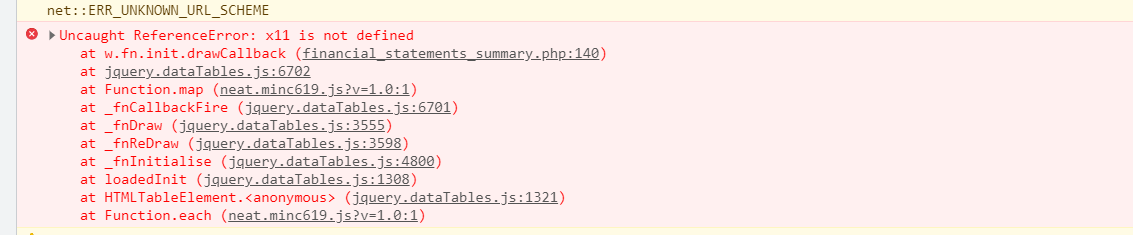# Row grouping and its subTotal for multiple level

I want to group multiple columns and also display the sub totals of each groups respectively using rowGroup extension

• Thanks for your response, but I need Multiple Group and its Multiple subtotal.

• Doesn't look like multiple groups are supported at this time:
https://datatables.net/forums/discussion/comment/122858/#Comment_122858

Kevin

• That is correct. Its something that is yet to be written.

Allan

• ok.Is there any other way to resolve my issue?

• Is there any other way to resolve my issue? Can anybody help pls?

• Yes, you could write the code for it if you wanted that feature. Its open source, so any contributions back would be warmly received.

Allan

• ok thanks for suggestions

• As I have resolved this issue using drawCallback function and without using rowGroup extension. Thanking you all for your valuable comments and suggestions.

• `````` drawCallback: function(settings) {
var api = this.api();
var rows = api.rows({ page: 'current' }).nodes();
var last = null;
var cols = api.row(0).data().length;
var totale = new Array();
totale['Totale'] = new Array();
var groupid = -1;
var subtotale = new Array();
var columns = x11;
var columns1 = x111 , s=0 ;
var colNo = [0,1,2];

var count= 0, svalue= [];

for (var c = 0; c < columns.length; c++) {
colNo = columns[c];
api.column(colNo, { page: 'current' }).data().each(function(group, i) {
if (last !== group) {
svalue.push(count);
groupid++;
\$(rows).eq(i).before(
'<tr class="group group-start"><td colspan="50">  ' + group + '</td></tr>'
);
last = group;
count= i;
}
else
{
count=count+1;
}

var val = api.row(api.row(\$(rows).eq(i)).index()).data();
\$.each(val, function(index2, val2) {
if(index2 == 4)
{
if (typeof subtotale[groupid] == 'undefined') {
subtotale[groupid] = new Array();
}
if (typeof subtotale[groupid][index2] == 'undefined') {
subtotale[groupid][index2] = 0;
}
var valore = Number(val2.replace(/[^\d]/g, '')*1);
subtotale[groupid][index2] = +parseFloat(subtotale[groupid][index2] + valore).toFixed(2);
}
});
});
}
if(svalue.length>groupid)
{
svalue.push(count);
groupid++;
}
for (var c = 0; c < svalue.length-1;) {
var colNo2 = columns[c];
api.column(colNo2, { page: 'current' }).data().each(function(group, i) {
var  subtd = '';
subtd = "Sum for "+group+" : "+ \$.fn.dataTable.render.number(',', '.', 0).display(subtotale[s]) ;
if(i == svalue[c+1])
{
console.log("groupgroup222", group , last);
\$(rows).eq(i).after(
'<tr class="group group-end"><td colspan="50">'+subtd+'</td></tr>');
s++;
c++;
}
});
}
}
``````
• This discussion has been closed.

• Hi @Rupesh2018,

Hope you're doing great !

Can you help me out in achieving this (see in image attached ) functionality using datatables

Thanks

• @Rupesh2018 Can I see your total code for this case. Thank you very much.

Hi @lovekiiz ,

Multi-level grouping is supported now - would that work for you?

Cheers,

Colin

• Hi @colin
For Multiple level grouping this is ok. but if we want aggregate function for the same multiple groups. This is not sufficient.

Why's that? We're happy to take a look, but as per the forum rules, please link to a test case - a test case that replicates the issue will ensure you'll get a quick and accurate response. Information on how to create a test case (if you aren't able to link to the page you are working on) is available here.

Cheers,

Colin

• @Rupesh2018

What is x11 and x111 in your code? I had put my desired row grouping column number.

Please have a look on attached image and let me know.